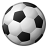# QlikView App Dev

Discussion Board for collaboration related to QlikView App Development.

cancel
Showing results for
Did you mean:Creator III

## Master calendar month number issue

Hi all,

I have a master calendar ranging from Jan-2017 to December-2018. I have generated month number for all the 24 months. The problem i am facing is that Max(MonthNumber) gives me Dec-18, but i want to point at the current month that is Jan-18.

Is there a way i can identify the month number of current month which i can use in set analysis?

Thanks!

1 Solution

Accepted SolutionsMVP

May be like this

Sum({<MonthYearField= {"\$(='<=' & Date(MonthStart(Today()), 'MMM-YY'))"}>}Sales)

13 RepliesMVP

May be like this

Date(MonthStart(Today()), 'MMM-YY')Creator III
Author

I am using the same thing for current month, and for previous months i am just subtracting from it and getting the required months. But consider the following scenario:

I need to know the

1. sum(sales) - Current month

2. sum(sales) - Previous month

3. sum(sales) - All the rest of the previous months.

The third point is where i am facing problemCreator

You could try this..

num(     month(<Date>)     )     ) // will return 1 for Jan 18

=Year (<Date>) *100+ num(month(<Date>)) // willl return 201801

Thanks D

🙂MVP

May be like this

Sum({<MonthYearField= {"\$(='<=' & Date(MonthStart(Today()), 'MMM-YY'))"}>}Sales)Creator

If you want current then use now() instead of <date>

You could try this..

num(     month(now())     )     ) // will return 1 for Jan 18

=Year (now()) *100+ num(month(now())) // willl return 201801

Thanks D

🙂Creator

Hi Arpit,

In all my reports with dates fields, I create indexes such as a master field called Month_index using below:

= (

Num( Year(Date) ) * 12 + Num( Month(Date) )

) // Creates month number for each number

-

(

Num( Year(Now()) ) * 12 + Num( Month(Now()) )

)// Creates month number for this month

This counts backwards from current month

0 = current month

-1 = last month

-2 = previous month

etc

means you can then use simple set analysis such as

sum(  Sales {\$<Month_index  = '0'>}) //current month

sum(  Sales {\$<Month_index  = '-1'>}) // previous month

sum(  Sales {\$<Month_index  = { '<-1'}>}) // All other previous months

Same can be applied to weeks and years etc...

Thanks

D

🙂Creator III
Author

Thank u so much Sunny. Its working just fine!Creator III
Author

Thanks for the reply Darrell. This one may also work fine!Creator III
Author

Is it possible to select month ranges here?

Like 0-4 months,5-6 months, where

0-Jan-18

1-Dec-17

2-Nov-17 and so on?